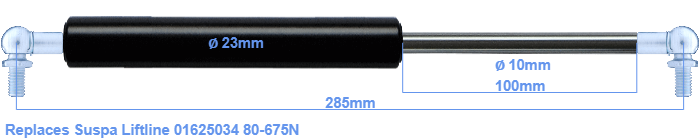# Replacement for Suspa Liftline 01625034 80-675N

\$35.92

Replacement gas spring for the Suspa Liftline 01625034 80-675 Newton. This fits a (possibly already present) ball with a diameter of 10mm. A corresponding ball is included. Brand: Stabilus Industry Line.

Please note:
The diameter of the tube of this replacement gas spring is 23mm instead of 22mm.
 Force Choose an option80 Newton100 Newton120 Newton140 Newton150 Newton160 Newton180 Newton200 Newton220 Newton240 Newton250 Newton260 Newton280 Newton300 Newton320 Newton340 Newton350 Newton360 Newton380 Newton400 Newton420 Newton440 Newton450 Newton460 Newton480 Newton500 Newton520 Newton540 Newton550 Newton560 Newton580 Newton600 Newton620 Newton640 Newton650 Newton660 Newton675 NewtonClear
This gas spring is also known as 16-4 016 25034, 16-4-148-100-A207-B207.The bore of the house of this replacement gas spring is 23 mm. The rod has a diameter of 10 mm. The stroke is 100 millimeter long. The total length of this replacement is exactly 285 mm. This is the total distance between the centers of the two mounting parts. Without the mounting parts this gas spring is 245 mm long (thread to thread). The force of this replacement gas spring is 80-675 Newton. Attention: this is not an official Suspa Liftline gas spring, but a replacement gas spring. This a gas spring with the brand Stabilus Industry Line. The force (80-675 N) and dimensions are close to identical to the original.
Category: